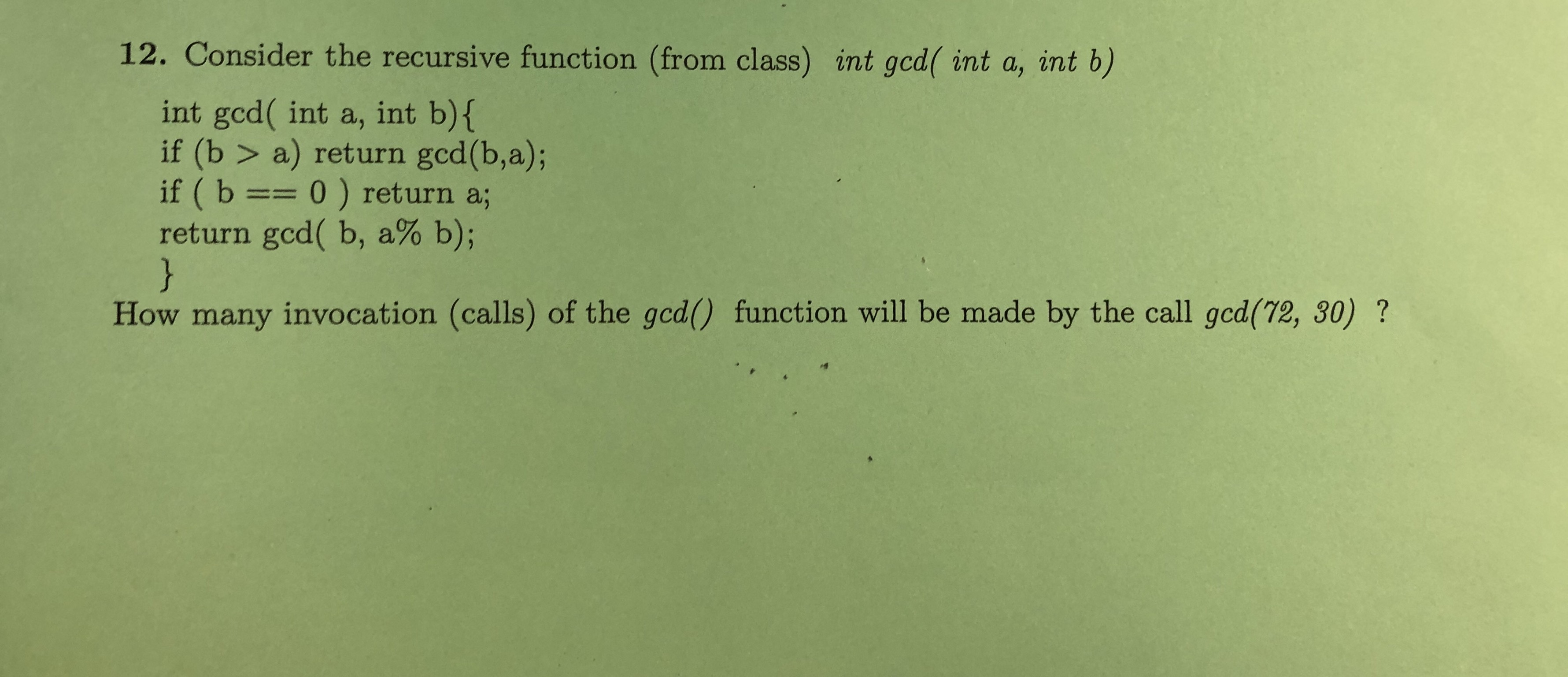# 12. Consider the recursive function (from class) int gcd( int a, int b)int gcd( int a, int b){if (b > a) return gcd(b,a);if ( b == 0 ) return a;return gcd( b, a% b);}How many invocation (calls) of the gcd() function will be made by the call gcd(72, 30) ?

Question
4 viewshelp_outlineImage Transcriptionclose12. Consider the recursive function (from class) int gcd( int a, int b) int gcd( int a, int b){ if (b > a) return gcd(b,a); if ( b == 0 ) return a; return gcd( b, a% b); } How many invocation (calls) of the gcd() function will be made by the call gcd(72, 30) ? fullscreen
check_circle

Step 1

Recursive function: A recursive function is a function that during its execution calls itself.

Code:

#include <iostream>

using namespace std;

//gcd function to calculate the gcd of two numbers

int gcd(int a, int b) {

if (b > a)

return gcd(b, a);

if (b == 0)

return a;

return gcd(b, a % b);

}

//main method

int main() {

//calling gcd function to find gcd of 72 and 30

cout << gcd(72, 30);

return 0;

}

Step 2

Output:

Output of above program is g...

### Want to see the full answer?

See Solution

#### Want to see this answer and more?

Solutions are written by subject experts who are available 24/7. Questions are typically answered within 1 hour.*

See Solution
*Response times may vary by subject and question.
Tagged in

### Programing Language